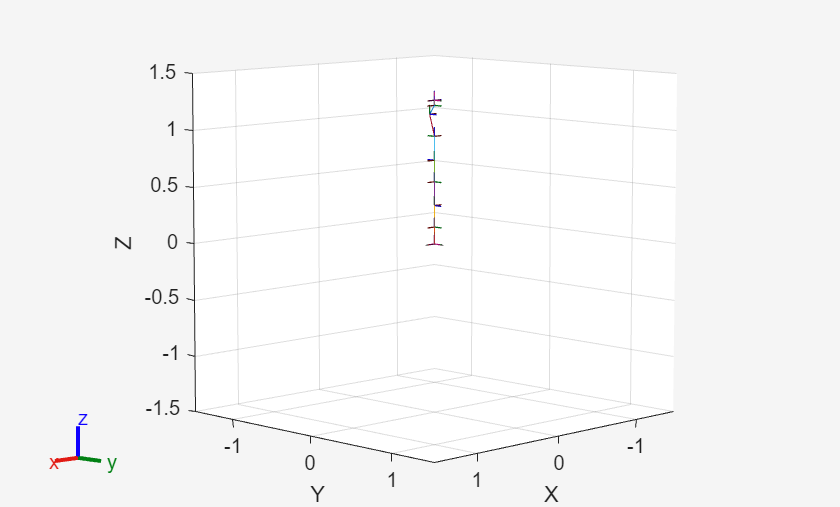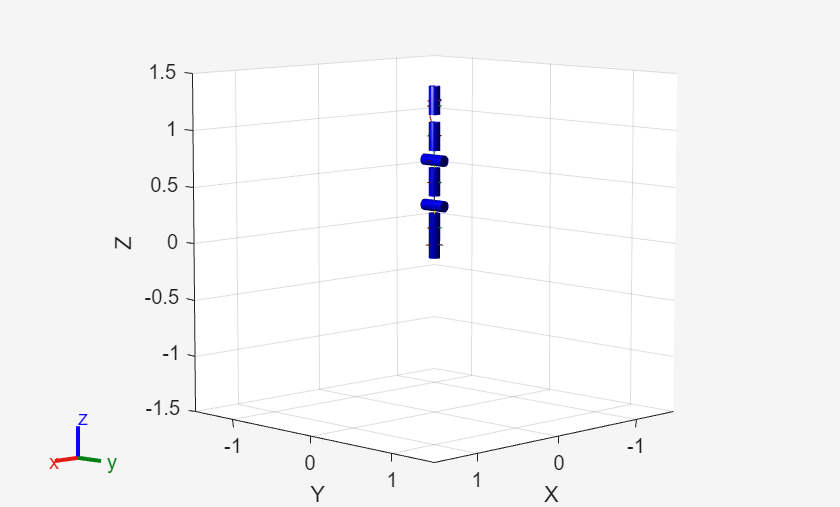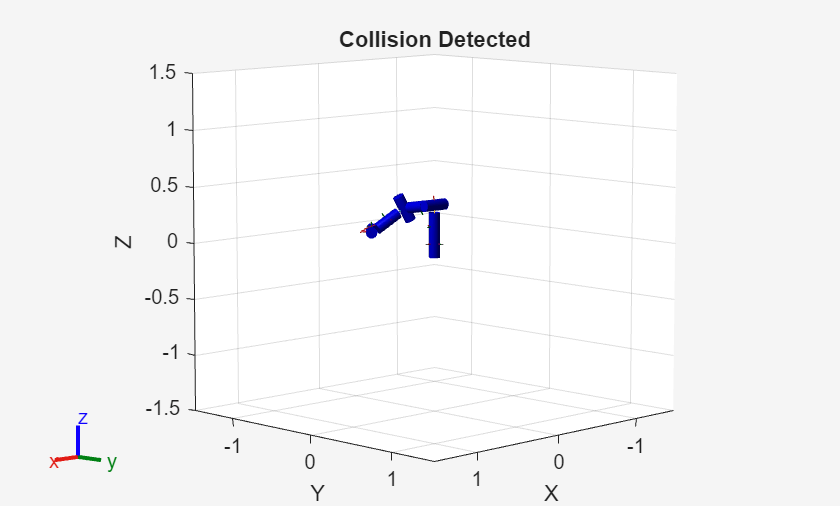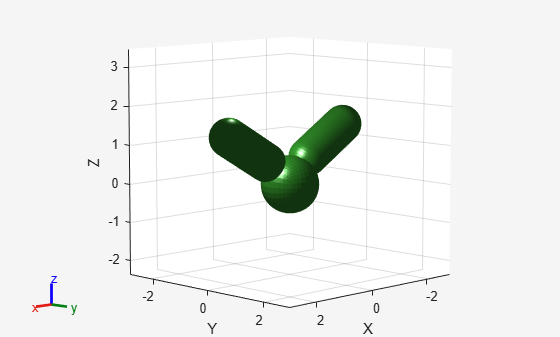# checkCollision

Check if robot is in collision

Since R2020b

## Syntax

``[isSelfColliding,selfSeparationDist,selfWitnessPts] = checkCollision(robot,config)``
``[isColliding,separationDist,witnessPts] = checkCollision(robot,config,worldObjects)``
``[___] = checkCollision(___,Name,Value)``

## Description

example

````[isSelfColliding,selfSeparationDist,selfWitnessPts] = checkCollision(robot,config)` checks if the specified rigid body tree robot model `robot` is in self-collision at the specified configuration `config`. Add collision objects to the rigid body tree robot model using the `addCollision` function. The `checkCollision` function also returns the closest separation distance `selfSeparationDist` and the witness points `selfWitnessPts` as points on each body.The function ignores adjacent bodies when checking for self-collisions.```
````[isColliding,separationDist,witnessPts] = checkCollision(robot,config,worldObjects)` checks if the specified rigid body tree robot model is in collision with itself or a specified set of collision objects in the world `worldObjects`.```
````[___] = checkCollision(___,Name,Value)` specifies additional options using one or more name-value pair arguments in addition to any of argument combinations from previous syntaxes.```

## Examples

collapse all

Load a robot model and modify the collision meshes. Clear existing collision meshes, add simple collision object primitives, and check whether certain configurations are in collision.

Load a preconfigured robot model into the workspace using the `loadrobot` function. This model already has collision meshes specified for each body. Iterate through all the rigid body elements and clear the existing collision meshes. Confirm that the existing meshes are gone.

```robot = loadrobot('kukaIiwa7','DataFormat','column'); for i = 1:robot.NumBodies clearCollision(robot.Bodies{i}) end show(robot,'Collisions','on','Visuals','off');```Iteratively add a collision cylinder to each body. Skip some bodies for this specific model, as they overlap and always collide with the end effector (body 10).

```collisionObj = collisionCylinder(0.05,0.25); for i = 1:robot.NumBodies if i > 6 && i < 10 % Skip these bodies. else addCollision(robot.Bodies{i},collisionObj) end end show(robot,'Collisions','on','Visuals','off');```Check for Collisions

Generate a series of random configurations. Check whether the robot is in collision at each configuration. Visualize each configuration that has a collision.

```figure rng(0) % Set random seed for repeatability. for i = 1:20 config = randomConfiguration(robot); isColliding = checkCollision(robot,config,'SkippedSelfCollisions','parent'); if isColliding show(robot,config,'Collisions','on','Visuals','off'); title('Collision Detected') else % Skip non-collisions. end end```This example shows how to change which rigid body pairs are skipped during self-collision checking in rigid body trees using the `SkippedSelfCollisions` name-value argument for `checkCollision`.

Serial Manipulator Robot

Load a serial manipulator robot represented as a two joint rigid body tree. Since this robot does not have collision geometries, add some primitive collision geometries.

```rbt2j = twoJointRigidBodyTree; P = [0.05 0.45]; % Geometry parameters for capsules T = trvec2tform([0.2 0 0]) * eul2tform([0 pi/2 0],"XYZ"); % Transformation parameters for capsules addCollision(rbt2j.Base,"cylinder",[0.075 0.1],trvec2tform([0 0 0.05])) addCollision(rbt2j.Bodies{1},"capsule",P,T) addCollision(rbt2j.Bodies{2},"capsule",P,T) addCollision(rbt2j.Bodies{3},"box",[0.2 0.05 0.2])```

Visualize the robot with collisions on.

`show(rbt2j,homeConfiguration(rbt2j),Collisions="on");`By default, `SkippedSelfCollisions` is `"parent"`, so self-collision checking skips collisions between parent and child bodies. Check the parent and child bodies of body2.

```body2 = rbt2j.Bodies{2}; rbt2j.BodyNames```
```ans = 1x3 cell {'body1'} {'body2'} {'tool'} ```
`body2.Parent.Name`
```ans = 'body1' ```
`body2.Children{1}.Name`
```ans = 'tool' ```

This means that `"body2"` is not checked for collisions against `"body1"` or `"tool"`.

List the body names of the robot. This shows that in the cell array, `"body2"`, which is stored at index 2, is adjacent to both `"body1"` at index 1 and `"tool"` at index 3. Because the skipped collision pairs have not changed, the `SkippedSelfCollisions` name-value argument has no effect on the self-collision checking result for this robot.

`rbt2j.BodyNames`
```ans = 1x3 cell {'body1'} {'body2'} {'tool'} ```

Run collision checking with both `SkippedSelfCollisions` options to verify that the `SkippedSelfCollisions` name-value argument returns the same result for this robot.

`checkCollision(rbt2j,homeConfiguration(rbt2j),SkippedSelfCollisions="parent")`
```ans = logical 0 ```
`checkCollision(rbt2j,homeConfiguration(rbt2j),SkippedSelfCollisions="adjacent")`
```ans = logical 0 ```

Parallel Manipulator Robot

Use the exampleHelperCreate2ArmRBT example helper to create a parallel robot comprised of two one-joint arms.

```rbt2arm = exampleHelperCreate2ArmRBT; show(rbt2arm,homeConfiguration(rbt2arm),Collisions="on"); axis padded```List the body names of the robot. The skipped body pairs formed by bodies of adjacent indices is similar to the serial manipulator but without body, `"tool"`.

`rbt2arm.BodyNames`
```ans = 1x2 cell {'body1'} {'body2'} ```

Check the parent of body1 and the parent of body2. Each body forms a parent-child relationship with the base, even though they are at adjacent indices.

`rbt2arm.Bodies{1}.Parent.Name`
```ans = 'base' ```
`rbt2arm.Bodies{2}.Parent.Name`
```ans = 'base' ```

Run collision checking with both `SkippedSelfCollisions` options.

`checkCollision(rbt2arm,homeConfiguration(rbt2arm),SkippedSelfCollisions="parent")`
```ans = logical 0 ```
`checkCollision(rbt2arm,homeConfiguration(rbt2arm),SkippedSelfCollisions="adjacent")`
```ans = logical 1 ```

As expected, when skipping parent-child body pairs during self collision checks, `checkCollision` finds no self collisions, but does find a self collision between the `"base"` and `"body2"` when skipping body pairs of adjacent indices.

## Input Arguments

collapse all

Rigid body tree robot model, specified as a `rigidBodyTree` object. To use the `checkCollision` function, the DataFormat property of the `rigidBodyTree` object must be either `'row'` or `'column'`.

Joint configuration of the rigid body tree, specified as an n-element numeric vector, where n is the number of nonfixed joints in the robot model. Each element of the vector is a specific joint position for a joint in the robot model.

Data Types: `single` | `double`

List of collision objects in the world, specified as a cell array of collision objects with any combination of `collisionBox`, `collisionCylinder`, `collisionSphere`, and `collisionMesh` objects. The function assumes that the `Pose` property of each object is relative to the base of the rigid body tree robot model.

### Name-Value Arguments

Specify optional pairs of arguments as `Name1=Value1,...,NameN=ValueN`, where `Name` is the argument name and `Value` is the corresponding value. Name-value arguments must appear after other arguments, but the order of the pairs does not matter.

Before R2021a, use commas to separate each name and value, and enclose `Name` in quotes.

Example: `'Exhaustive','on'` enables exhaustive checking for collisions and causes the function to calculate all separation distances and witness points.

Exhaustively check for all collisions, specified as the comma-separated pair consisting of `'Exhaustive'` and `'on'` or `'off'`. By default, the function finds the first collision and stops, returning the separation distances and witness points for incomplete checks as `Inf`.

If this name-value pair argument is specified as `'on'`, the function instead continues checking for collisions until it has exhausted all possibilities.

Data Types: `char` | `string`

Skip checking for robot self-collisions, specified as the comma-separated pair consisting of `'IgnoreSelfCollision'` and `'on'` or `'off'`. When this argument is enabled, the function ignores collisions between the collision objects of the rigid body tree robot model bodies and other collision objects of the same model or its base.

This name-value pair argument affects the size of the `separationDist` and `witnessPts` output arguments.

Data Types: `char` | `string`

Body pairs skipped for checking self-collisions, specified as either `"parent"` or `"adjacent"`:

• `"parent"` — Skip collision checking between child and parent bodies.

• `"adjacent"` — Skip collision checking between bodies on adjacent indices.

Data Types: `char` | `string`

## Output Arguments

collapse all

Self Collisions

Robot configuration is in self-collision returned as a logical `0` (`false`) or `1` (`true`). If the function returns a value of `true` for this argument, that means that one of the rigid body collision objects is touching another collision object in the robot model. Add collision objects to your rigid body tree robot model using the `addCollision` function.

Data Types: `logical`

Minimum separation distance between the bodies of the robot, returned as an (m+1) -by-(m+1) matrix, where m is the number of bodies. The final row and column correspond to the robot base. Units are in meters.

If a pair is in collision, the function returns the separation distance for the associated element as `NaN`.

Data Types: `double`

Witness points between the robot bodies including the base, returned as an 3(m+1)-by-2(m+1) matrix, where m is the number of bodies. Witness points are the points on any two bodies that are closest to one another for a given configuration. The matrix takes the form:

The matrix is divided into 3-by-2 sections that represent the xyz-coordinates of witness point pairs in the form:

`$\left[\begin{array}{cc}{x}_{1}& {x}_{2}\\ {y}_{1}& {y}_{2}\\ {z}_{1}& {y}_{2}\end{array}\right]$`

Each section corresponds to a separation distance in the `selfSeparationDist` output matrix. Use these equations to determine where the section of the `selfWitnessPts` matrix that corresponds to a specific separation distance begins:

`${W}_{r}=3{S}_{r}-2$`
`${W}_{c}=2{S}_{c}-1$`

Where (Sr,Sc) is the index of a separation distance in the separation distance matrix and (Wr,Wc) is the index in the witness point matrix at which the corresponding witness points begin.

If a pair is in collision, the function returns each coordinate of the witness points for that element as `NaN`.

Data Types: `double`

World Collisions

Robot configuration is in collision, returned as a two-element logical vector. The first element indicates whether the robot is in self-collision. The second element indicates whether the robot model is in collision with any world objects.

Data Types: `logical`

Minimum separation distance between the collision objected, returned as an (m+1)-by-(m+w+1) matrix, where m is the number of bodies and w is the number of world objects. The first m rows correspond to the robot bodies, where the (m+1)th row or column index corresponds to the base. The remaining w columns correspond to the world objects.

The matrix is divided into 3-by-2 sections that represent the xyz-coordinates of witness point pairs in the form:

`$\left[\begin{array}{cc}{x}_{1}& {x}_{2}\\ {y}_{1}& {y}_{2}\\ {z}_{1}& {y}_{2}\end{array}\right]$`

Each section corresponds to a separation distance in the `separationDist` output matrix. Use these equations to determine where the section of the `witnessPts` matrix that corresponds to a specific separation distance begins:

`${W}_{r}=3{S}_{r}-2$`
`${W}_{c}=2{S}_{c}-1$`

Where (Sr,Sc) is the index of a separation distance in the separation distance matrix and (Wr,Wc) is the index in the witness point matrix at which the corresponding witness points begin.

If a pair is in collision, the function returns each coordinate of the witness points for that element as `NaN`.

If a pair is in collision, the function returns the separation distance as `NaN`.

#### Dependencies

If you specify the `'IgnoreSelfCollision'` name-value pair argument as `'on'`, then the matrix does not contain values for the distances between any given body and other bodies in the robot model.

Data Types: `double`

Witness points between collision objects, specified as a 3(m+1)-by-2(m+w+1) matrix, where `m` is the number of bodies and `w` is the number of world objects. Witness points are the points on any two bodies that are closest to one another for a given configuration. The matrix takes the form:

```[Wr1_1 Wr1_2 ... Wr1_(N+1) Wo1_1 Wo1_2 ... W1_M; Wr2_1 Wr2_2 ... Wr2_(N+1) Wo2_1 Wo2_2 ... W2_M; . . . . . . . . . . . . . . . . . . . . . . . . Wr(N+1)_1 Wr(N+1)_2 ... Wr(N+1)_(N+1) Wo(N+1)_1 Wo(N+1)_2 ... W(N+1)_M]```

Each element in the above matrix is a 2-by-3 matrix that gives the nearest `[x y z]` points on the two corresponding bodies or world objects. The final row and column correspond to the robot base.

If a pair are in collision, witness points for that element are returned as `NaN(3,2)`.

#### Dependencies

If the `"IgnoreSelfCollision"` name-value pair is set to `"on"`, then the matrix contains no `Wr` elements.

Data Types: `double`

## Version History

Introduced in R2020b

expand all